Examples

Chapter 15 Class 10 Probability
Serial order wise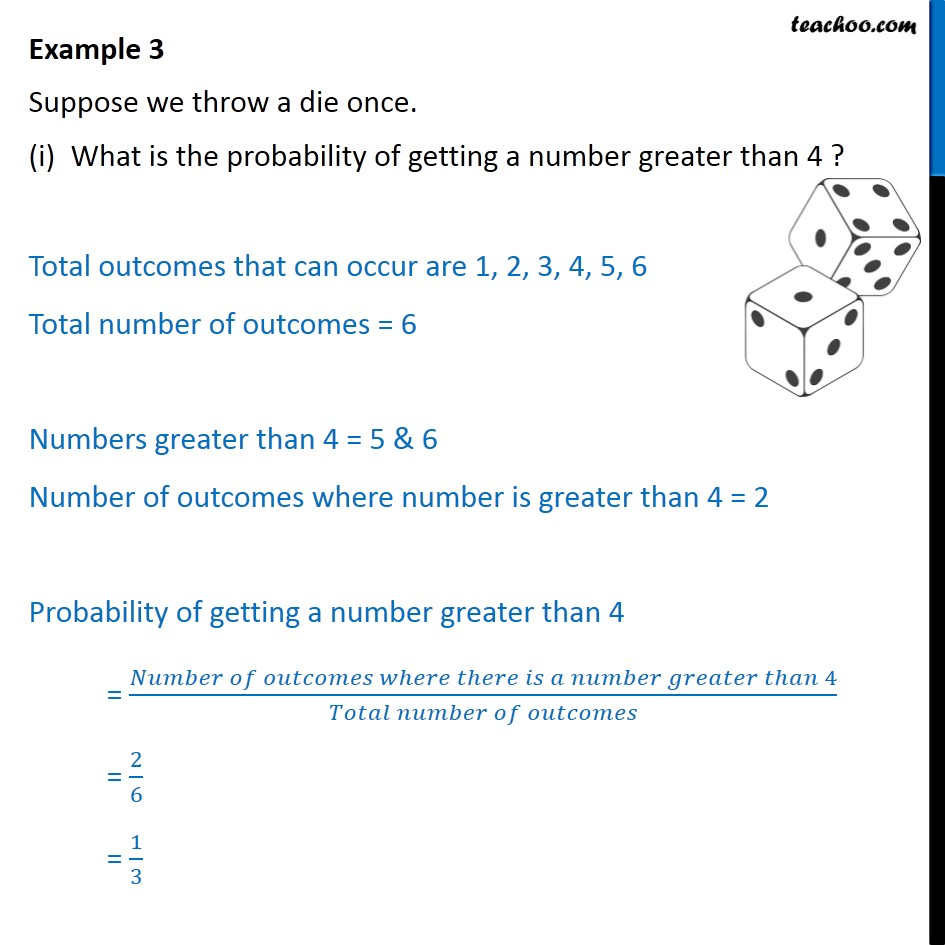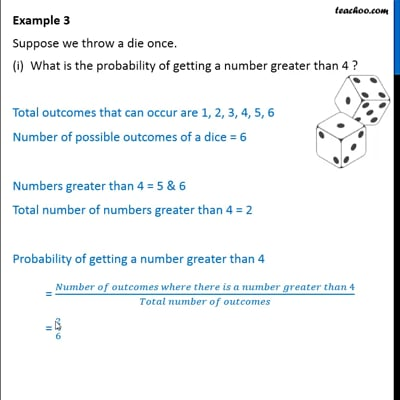This video is only available for Teachoo black users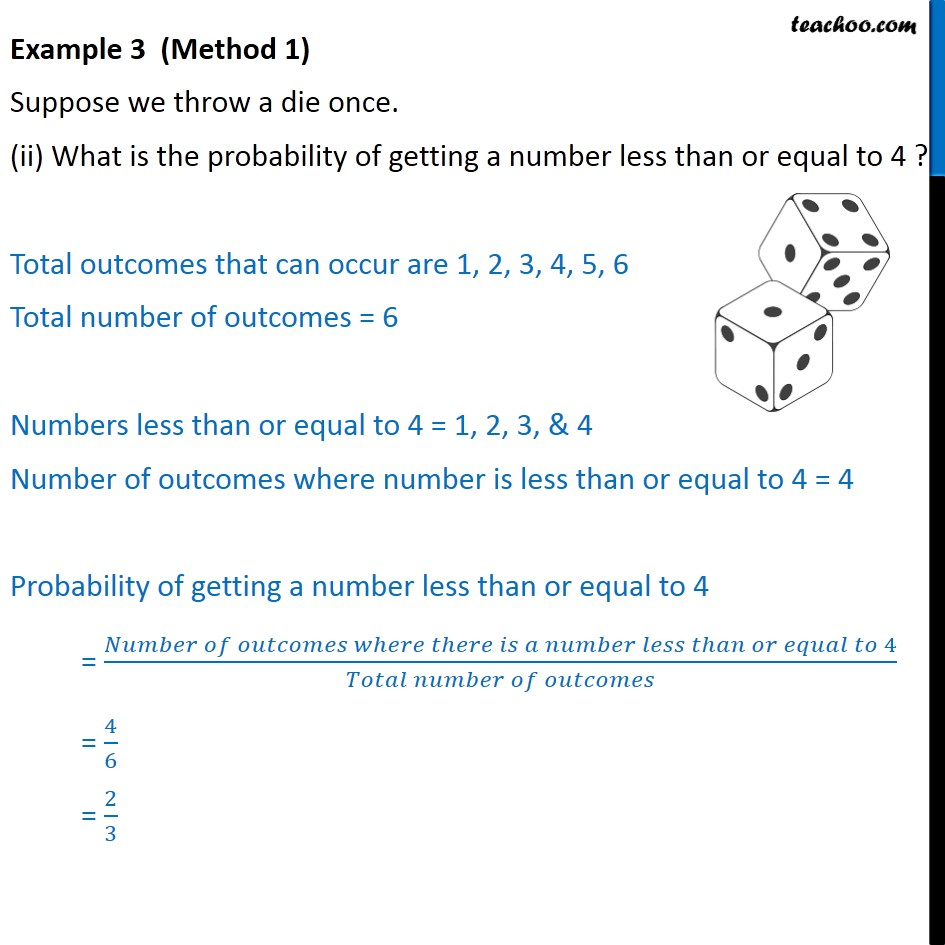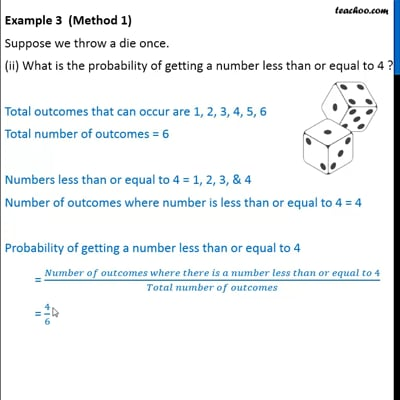This video is only available for Teachoo black users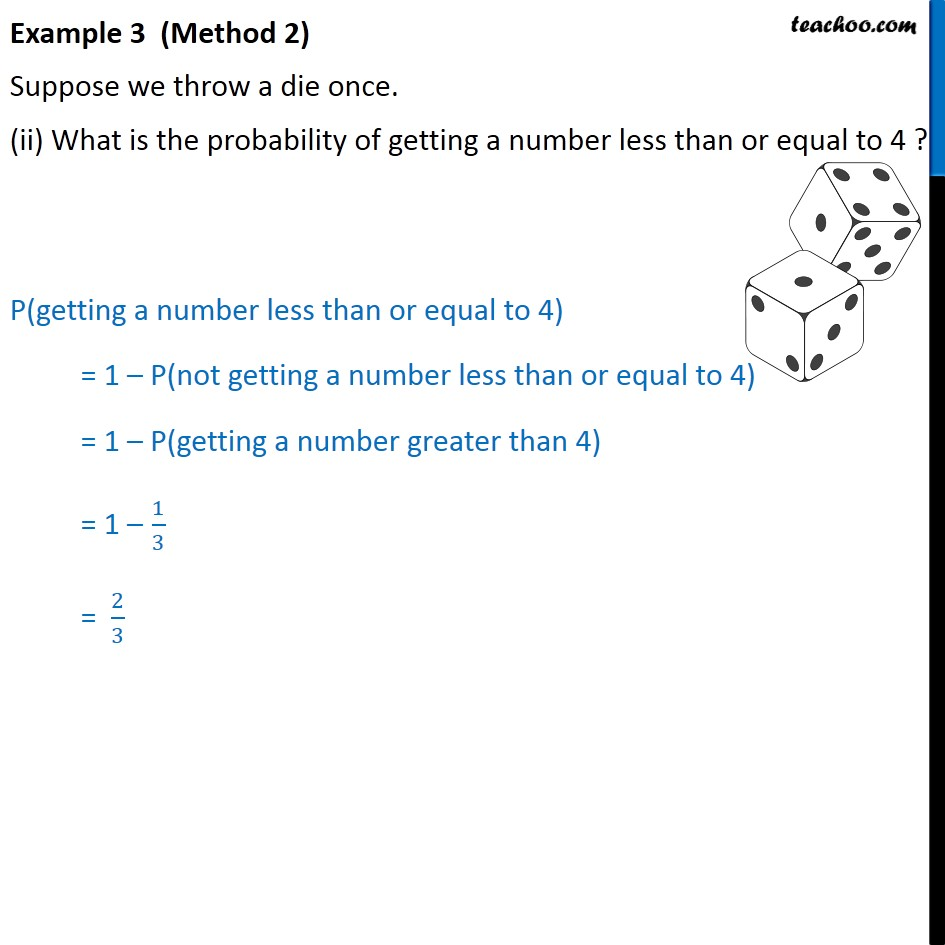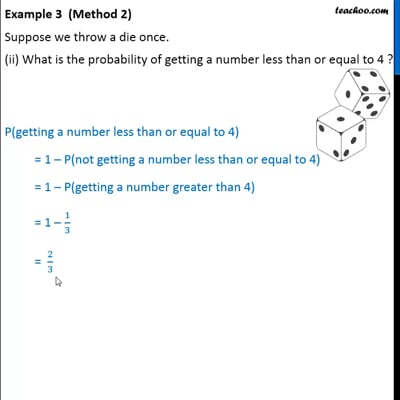This video is only available for Teachoo black users

Maths Crash Course - Live lectures + all videos + Real time Doubt solving!

### Transcript

Example 3 Suppose we throw a die once. What is the probability of getting a number greater than 4 ? Total outcomes that can occur are 1, 2, 3, 4, 5, 6 Total number of outcomes = 6 Numbers greater than 4 = 5 & 6 Number of outcomes where number is greater than 4 = 2 Probability of getting a number greater than 4 = (𝑁𝑢𝑚𝑏𝑒𝑟 𝑜𝑓 𝑜𝑢𝑡𝑐𝑜𝑚𝑒𝑠 𝑤ℎ𝑒𝑟𝑒 𝑡ℎ𝑒𝑟𝑒 𝑖𝑠 𝑎 𝑛𝑢𝑚𝑏𝑒𝑟 𝑔𝑟𝑒𝑎𝑡𝑒𝑟 𝑡ℎ𝑎𝑛 4)/(𝑇𝑜𝑡𝑎𝑙 𝑛𝑢𝑚𝑏𝑒𝑟 𝑜𝑓 𝑜𝑢𝑡𝑐𝑜𝑚𝑒𝑠) = 2/6 = 1/3 Example 3 (Method 1) Suppose we throw a die once. (ii) What is the probability of getting a number less than or equal to 4 ? Total outcomes that can occur are 1, 2, 3, 4, 5, 6 Total number of outcomes = 6 Numbers less than or equal to 4 = 1, 2, 3, & 4 Number of outcomes where number is less than or equal to 4 = 4 Probability of getting a number less than or equal to 4 = (𝑁𝑢𝑚𝑏𝑒𝑟 𝑜𝑓 𝑜𝑢𝑡𝑐𝑜𝑚𝑒𝑠 𝑤ℎ𝑒𝑟𝑒 𝑡ℎ𝑒𝑟𝑒 𝑖𝑠 𝑎 𝑛𝑢𝑚𝑏𝑒𝑟 𝑙𝑒𝑠𝑠 𝑡ℎ𝑎𝑛 𝑜𝑟 𝑒𝑞𝑢𝑎𝑙 𝑡𝑜 4)/(𝑇𝑜𝑡𝑎𝑙 𝑛𝑢𝑚𝑏𝑒𝑟 𝑜𝑓 𝑜𝑢𝑡𝑐𝑜𝑚𝑒𝑠) = 4/6 = 2/3 Example 3 (Method 2) Suppose we throw a die once. (ii) What is the probability of getting a number less than or equal to 4 ? P(getting a number less than or equal to 4) = 1 – P(not getting a number less than or equal to 4) = 1 – P(getting a number greater than 4) = 1 – 1/3 = 2/3